The t-test is a very useful test that compares one variable (perhaps blood pressure) between two groups. T-tests are called t-tests because the test results are all based on t-values. T-values are an example of what statisticians call test statistics. A test statistic is a standardized value that is calculated from sample data during a hypothesis test. It is used to determine whether there is a significant difference between the means of two groups. With all inferential statistics, we assume the dependent variable fits a normal distribution. When we assume a normal distribution exists, we can identify the probability of a particular outcome. The procedure that calculates the test statistic compares your data to what is expected under the null hypothesis. There are several SAS Studio tasks that include options to test this assumption. Let's use the t-test task as an example.

You start by selecting:

On the DATA tab, select the Cars data set in the SASHELP library. Next request a Two-sample test, with Horsepower as the Analysis variable and Cylinders as the Groups variable. Use a filter to include only 4- or 6-cylinder cars. It should look like this: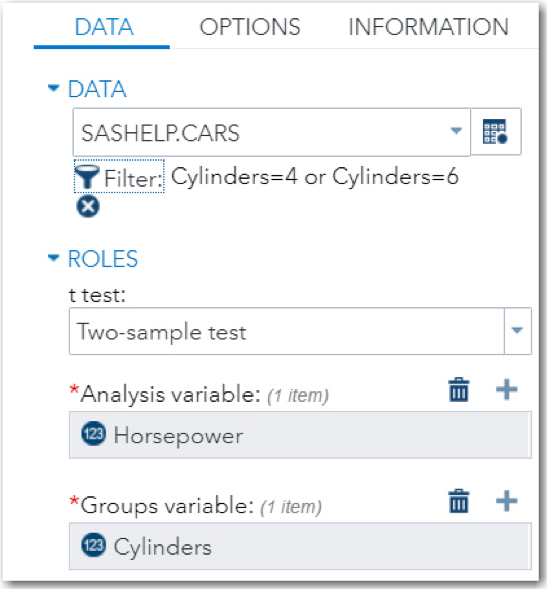On the OPTIONS tab, check the box for Tests for normality as shown below.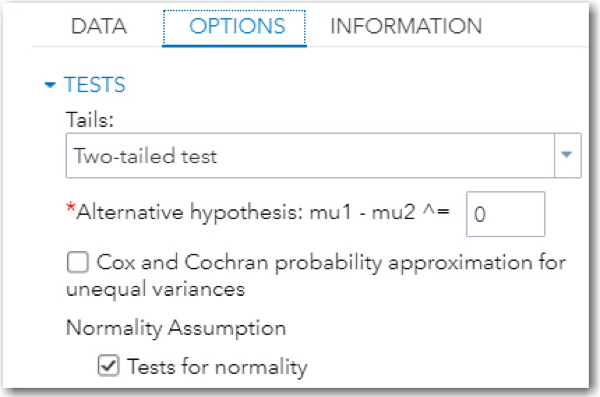All the tests for normality for both 4-cylinder and 6-cylinder cars reject the null hypothesis that the data values come from a population that is normally distributed. (See the figure below.)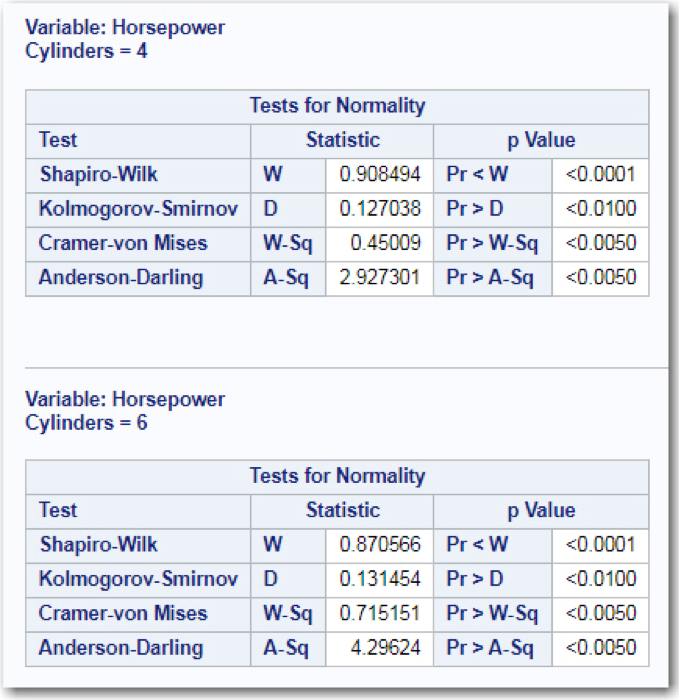### Should you abandon the t-test results and run a nonparametric test analysis such as a Wilcoxon Rank Sum test that does not require normal distributions?

This is the point where many people make a mistake. You cannot simply look at the results of the tests for normality to decide if a parametric test is valid or not. Here is the reason: When you have large sample sizes (in this data set, there were 136 4-cylinder cars and 190 6-cylinder cars), the tests for normality have more power to reject the null hypothesis and often result in p-values less than .05. When you have small sample sizes, the tests for normality will not be significant unless there are drastic departures from normality. It is with small sample sizes where departures from normality are important.

The bottom line is that the tests for normality often lead you to make the wrong decision. You need to look at the distributions and decide if they are somewhat symmetrical. The central limit theory states that the sampling distribution of means will be normally distributed if the sample size is sufficiently large. "Sufficiently large" is a judgment call. If the distribution is symmetrical, you may perform a t-test with sample sizes as small as 10 or 20.

The figure below shows you the distribution of horsepower for 4- and 6-cylinder cars.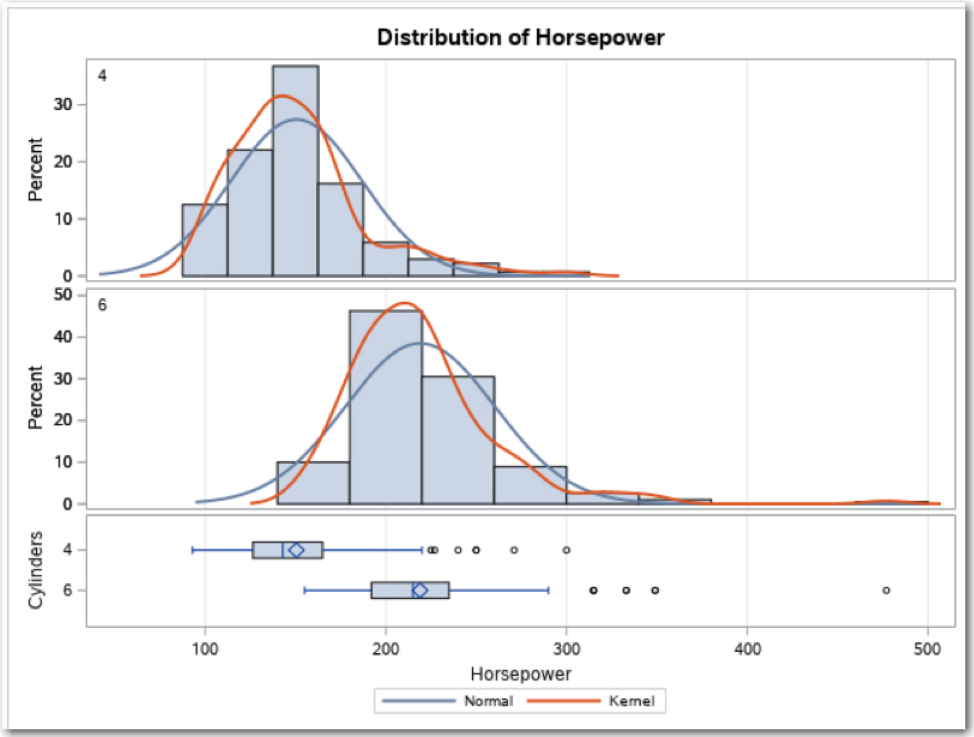With the large sample sizes in this data set, you should feel comfortable in using a t-test. The results, shown below, are highly significant.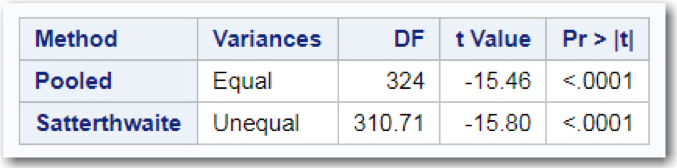If you are in doubt of your decision to use a parametric test, feel free to check the box for a nonparametric test on the OPTIONS tab. Running a Wilcoxon Rank Sum test (a nonparametric alternative to a t-test), you also find a highly significant difference in horsepower between 4- and 6-cylinder cars. (See the figure below.)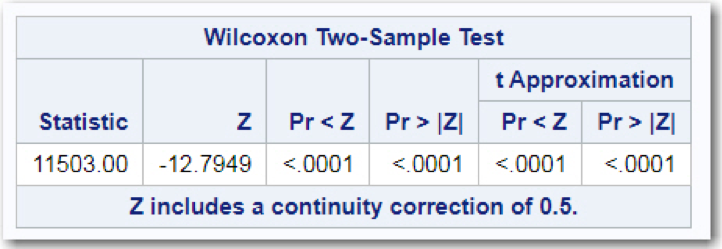You can read more about assumptions for parametric tests in my new book, A Gentle Introduction to Statistics Using SAS Studio.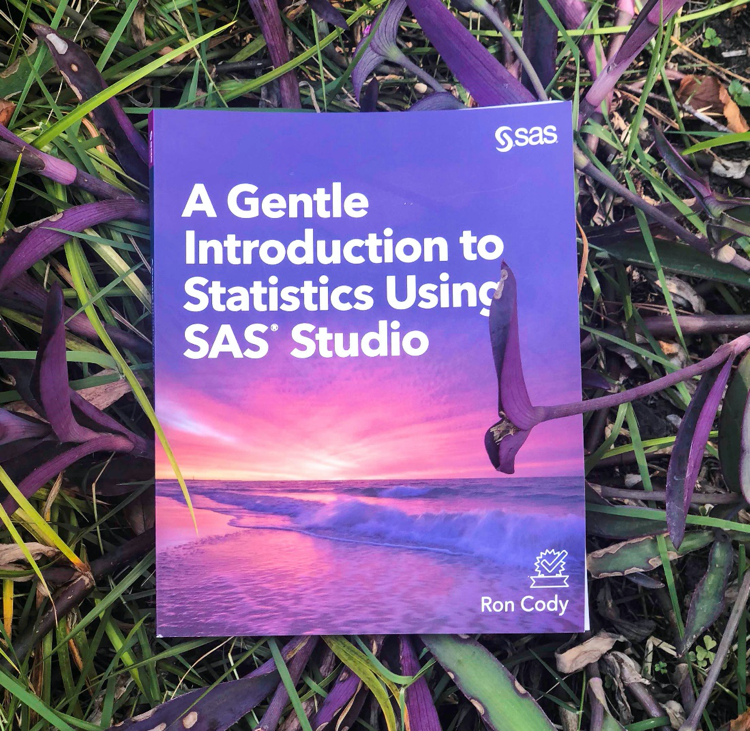For a sneak preview check out the free book excerpt. You can also learn more about SAS Press, check out the up-and-coming titles, and receive exclusive discounts. To do so, make sure to subscribe to the newsletter.

Testing the Assumption of Normality for Parametric Tests was published on SAS Users.